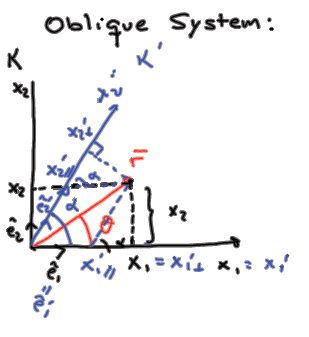# Covariant and Contravariant components in Oblique System

tylerscott

## Homework StatementIn the oblique coordinate system K' defined in class the position vector r′ can be written as:
$r'=a\hat{e'}_{1}+b\hat{e'}_{2}$

Are a and b the covariant (perpendicular) or contravariant (parallel) components of r′? Why? Give an explanation based on vectors’ properties and another based on tensors’ properties.

## Homework Equations

$\hat{e'}_1=\hat{e}_1$
$\hat{e'}_2=e_1cos(\alpha )+e_2sin(\alpha )$

${x'}_{1\perp}={x}_1$
${x'}_{2\perp}=x_1cos(\alpha )+x_2sin(\alpha )$

## The Attempt at a Solution

My best effort is that a and b are the covariant components of r' since they are the perpendicular projects of r' onto the $\hat{e'}_1$ and $\hat{e'}_2$ basis vectors, so they're essentially equivalent to ${x'}_{1\perp}$ and ${x'}_{2\perp}$. So I think this would be my vector solution for the problem, but I don't know exactly how to represent it. As for the an explanation based on the tensor's properties, I don't even know where to start...

Last edited:

## Answers and Replies

Muphrid
If you used the perpendicular projections onto the axes with the basis vectors given, would you recover the point $r$? It doesn't look like that to me. You'd end up way out above and to the right.

tylerscott
Ah, I see. I have been struggling with this problem for the past couple days and think I have developed a rough proof:
a and b are contravariant components of r' since they both give the magnitude of the vectors that add to r'. We found that:
$\hat{e'}_{1}=\hat{e}_{1}$
$\hat{e'}_{2}=\hat{e}_{1}cos(\alpha)+\hat{e}_{2}sin(\alpha)$
And
$x'_{1\parallel}=x_{1}-cot(\alpha)x_{2}$
$x'_{2\parallel}=x_{2}csc(\alpha)$

Making the substitutions into $r'=a\hat{e'}_{1}+b\hat{e'}_{2}$:
$r'=(x_{1}-cot(\alpha)x_{2}) \hat{e}_{1}+(x_{2}csc({\alpha}))(\hat{e}_{1}cos( \alpha)+\hat{e}_{2}sin(\alpha))$
We get
$r'={x}_{1}\hat{e}_{1}+{x}_{2}\hat{e}_{2}$

So I think this is good for the vector explanation. Any advice for the tensor representation?

Last edited:
Muphrid
Yeah, they should be contravariant components, but to be honest, when the question says "give an explanation based on the tensors' properties," I'm not even clear on which tensors are being talked about.

tylerscott
Maybe I'll have to present that to the professor. I thought that maybe she just wanted the same proof, but using Einstein notation for the matrices that make the transformation.

Mentor
e1' and e2' are unit vectors along the coordinate directions of the primed coordinate system. e'1 is the partial derivative of the arbitrary position vector r with respect to x1' and e'2 is the partial derivative of the arbitrary position vector r with respect to x2', where I have used a more suggestive notation here for "parallel" x primes. Thus,

r = x1'e1' + x2'e2'

Therefore, a = x1' and b = x2'. These are the contravariant components.